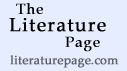# Charles Dickens: The Old Curiosity Shop

## Table of Contents

The Old Curiosity Shop (Fiction, 1841, 618 pages)

This title is not on Your Bookshelf. [Add to Shelf] (0 / 10 books on shelf)
 10. CHAPTER 10   11. CHAPTER 11   12. CHAPTER 12   13. CHAPTER 13   14. CHAPTER 14   15. CHAPTER 15   16. CHAPTER 16   17. CHAPTER 17   18. CHAPTER 18   19. CHAPTER 19   20. CHAPTER 20   21. CHAPTER 21   22. CHAPTER 22   23. CHAPTER 23   24. CHAPTER 24   25. CHAPTER 25 26. CHAPTER 26   27. CHAPTER 27   28. CHAPTER 28   29. CHAPTER 29   30. CHAPTER 30   31. CHAPTER 31   32. CHAPTER 32   33. CHAPTER 33   34. CHAPTER 34   35. CHAPTER 35   36. CHAPTER 36   37. CHAPTER 37   38. CHAPTER 38   39. CHAPTER 39   40. CHAPTER 40   41. CHAPTER 41   42. CHAPTER 42   43. CHAPTER 43   44. CHAPTER 44   45. CHAPTER 45   46. CHAPTER 46   47. CHAPTER 47   48. CHAPTER 48   49. CHAPTER 49   50. CHAPTER 50 51. CHAPTER 51   52. CHAPTER 52   53. CHAPTER 53   54. CHAPTER 54   55. CHAPTER 55   56. CHAPTER 56   57. CHAPTER 57   58. CHAPTER 58   59. CHAPTER 59   60. CHAPTER 60   61. CHAPTER 61   62. CHAPTER 62   63. CHAPTER 63   64. CHAPTER 64   65. CHAPTER 65   66. CHAPTER 66   67. CHAPTER 67   68. CHAPTER 68   69. CHAPTER 69   70. CHAPTER 70   71. CHAPTER 71   72. CHAPTER 72   73. CHAPTER 73

 Search for text within this title:
(c) 2003-2012 LiteraturePage.com and Michael Moncur. All rights reserved.
For information about public domain texts appearing here, read the copyright information and disclaimer.